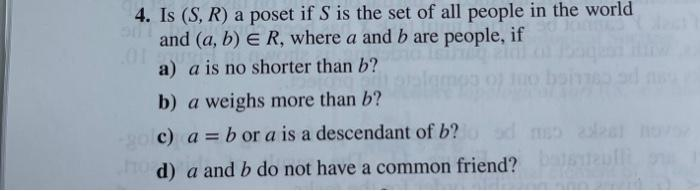# Question Solved1 Answer4. Is (S, R) a poset if S is the set of all people in the world and (a, b) E R, where a and b are people, if a) a is no shorter than b? b) a weighs more than b? c) a = b or a is a descendant of b? d) a and b do not have a common friend?Transcribed Image Text: 4. Is (S, R) a poset if S is the set of all people in the world and (a, b) E R, where a and b are people, if a) a is no shorter than b? b) a weighs more than b? c) a = b or a is a descendant of b? d) a and b do not have a common friend?
More
Transcribed Image Text: 4. Is (S, R) a poset if S is the set of all people in the world and (a, b) E R, where a and b are people, if a) a is no shorter than b? b) a weighs more than b? c) a = b or a is a descendant of b? d) a and b do not have a common friend?